# Letter and Symbol Series

Letter and Symbol Series are a sequential order of letters, numbers or both arranged such a way that each term in the series is obtained according to some specific rules. These rules can be based on mathematical operations, place of letters in alphabetical order etc.

Letter series is a logical arrangement of letters of English alphabet arranged in a specified pattern.

In this section, a series of letters, groups of letters or combinations of letters and numbers is given. Each group or single element is called term. The terms of the series form a certain pattern. Candidates are required to identify this pattern and find the missing term(s) in the given series which will satisfy the pattern.

## Types of Letter Series

There are mainly three types of letter series.
They are as follows

Type #1: Alphabet Series

In this type, letters of English alphabet are arranged in a particular pattern like reverse order of letters, skipping of letters, position of letters in alphabetical order etc.

Example 1: Find the next term in the series.

A, D, G, J, ?

(a) K    (b) L    (c) M    (d) N

Solution:  (c) Pattern of the series is a shown below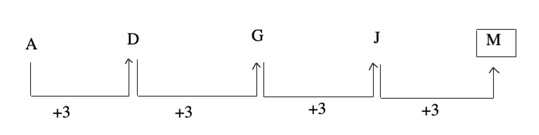Every next term of the series is next third letter of the alphabets (according to position).
Hence, the missing term is M.

Example 2: Find the next two terms of the given series.

Z, S, W, O, T, K, Q, G, ?, ?

(a) N, C        (b) N, D
(c) O, C        (d) O, D

Solution:  (a) The given series is a combination of two series.

I. Z, W, T, Q, ?   and   II. S, O, K, G, ?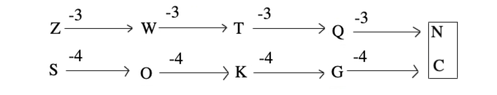Hence, the missing letters are N and C.

Example 3: Find the missing term in the given series.

ABP, CDQ, EFR, ?

(a) GHS        (b)GHT
(c) HGS        (d)GHR

Solution:  (a) The given series is a combination of three series.

I.   A, C, E, ?   and   II. B, D, F, ?
III. P, Q, R, ?

Now,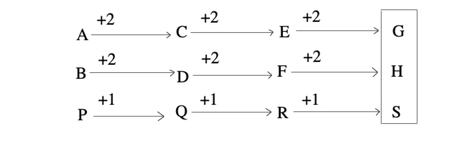Hence, the missing term is GHS.

Example 4: Find the missing term in the given series.

AB, DEF, HIJK, ?, STUVWX

(a) LMNO        (b) LMNOP
(c) MNOPQ        (d)QRSTU

Solution:  (c) Pattern of the series is as shown below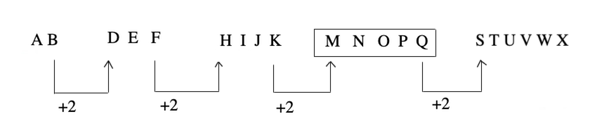The number of letters in the terms of given series increases by one at each step. The first letter of each term is two steps ahead of the last letter of the preceding term. However, each term consists of consecutive letters in order.

Hence, the missing term is MNOPQ.

Type #2: Continuous Pattern Series

In this type, a series of small/capital letters are given which follow a particular pattern.
However, some letters are missing from the series. The series follows a specific pattern and candidates are required to find the letters which should come in place of the blank spaces or question marks.

Directions (Example Nos. 5-6) These questions are based on the letter series. In each of these series, some of the letters are missing. Select the correct alternatives.

Example 5: abca_bcaab_ca_bbc_a

(a) ccaa        (b) bbaa
(c) abac        (d) abba

Solution:  (c) Pattern of the series is as shown below

abc / aabc / aabbc / aabbcc / a => abac

Hence, the missing letters are abac.

Example 6: _cb_ca_bacb_ca_bac_d

(a) badddb        (b) bbbddd
(c) addddb        (d) addbbb

Solution:  (c) Pattern of the series is as shown below

acbd / cadb / acbd / cadb / acbd  =>  addddb

The sequence is made of repetition of two blocks 'acbd' and 'cadb' alternatively.

Hence, the missing letters are addddb.

Type #3: Mixed Series

[Alpha-numeric Series]

In this type, the series is based on the combination of both the letters and numbers.
Each term in the series follows a certain pattern based on either the alphabetical position of the letters or the numbers in different correlation.

Example 7: Replace the question mark (?) in the following series with suitable option.

C-3, E-5, G-7, I-9, ?, ?

(a) M-18, K-14

(b) K-11, M-13

(c) X-24, M-21

(d) O-15, X-24

Solution:  (b) Pattern of the series is as shown below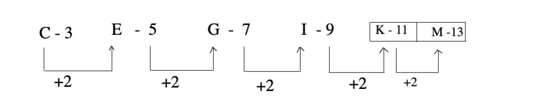In this series, every letter is given its alphabetical position and one letter is skipped in between each term.

Hence, the missing terms are K-11, M-13.

Example 8: What comes in place of question mark (?) in the series given below?

P3C, R5F, T8I, V12L, ?

(a) Y17O

(b) X16M

(c) X17O

(d) X16O

Solution:  (c) Pattern of the series is as shown below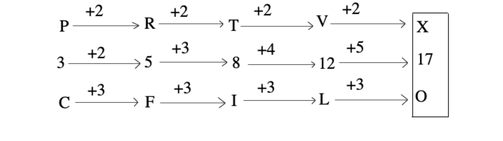Hence, the missing term is X17O

## Alphabetical Series Tricks

A B C D E F G H I J K L M N O P Q R S T U V W X Y Z

1. THE ALPHABET: There are 26 letters in English alphabets.

The first half of alphabet series is from A to M and the half is from N to Z.

A-M – 1-13 (First Alphabetical Half)

N-Z –14-26 (Second Alphabetical Half)

Hence So if you remember these intervals,It would be helpful in solving Letter/Alphabet Series problems.

2. Intervals like: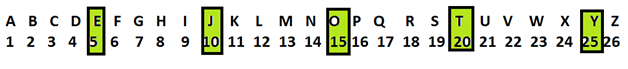I. EJOTY: To make it easier there is a trick called EJOTY (i.e From left to Right) by which you can simply locate the position of letter without much effort.

E      J      O      T         Y

5    10    15     20        25

Suppose we want to find the place of the 20th letter from the left side. And you know 20th letter is T, so now, you only have to find a letter which is two positions ahead of T, which is V.

II. VQLGB: It is similar to EJOTY but this is in reverse order i.e from right to left.

V      Q      L      G         B

5      10   15    20       25

By these simple tricks, you can instantly find the position of any letter either from the left side or the right side without much efforts.This trick will help you to solve basic questions on alphabet series.

3. Most of the questions from Alphabetic series in the exams will be from the right side as we are habitual of counting from the left side i.e A,B,C,D… But before we proceed here are few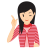Few Important things to Remember

i. From your left implies left to right.

ii. To the right implies from left to right.

iii. To the left implies from right to left.

For example, Let's find the place of a letter from right side which is the 14th from the left side.

## TIPS on cracking Reasoning Questions on Letter and Symbol Series

Tip #1: Break the complex letter series / alpha numeric series into simpler ones and solve them individually

Question: What should come in the place of question mark (?) in the alpha numeric series?

A2I, E4J, I8K, O16L, ?

Solution:

Pattern of the series as shown below

First letter of each term is a series of consecutive vowels, numbers are moved as 2, 22, 23, 24, 25 and third letter of each term is increasing consecutively.

Hence, the missing term is U32M.

Question: What should come in the place of question mark (?) in the alpha numeric series?

9Z-20, 11A-18, 13Y-16, 15B-14, ?

Solution:

Pattern of the series as shown below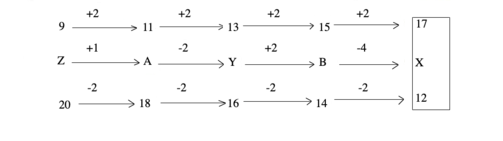Hence, the missing term is17X12.

Question: Which of the following terms follows the trend of the given list?

PRRQPQPQ, PQRRPQPQ, PQPRRQPQ,
PQPQRRPQ, PQPQPRRQ, ________.

Solution:

Pattern of the series as shown below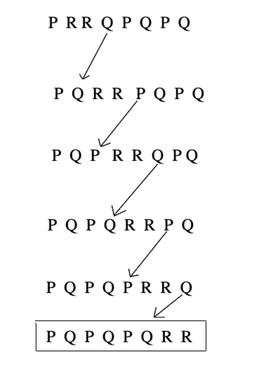Hence, the missing term is PQPQPQRR.

Tip #2: Identify the changes happening in the successive patterns of a symbol series.

Question: Look carefully at the sequence of symbols to find the missing pattern.

Solution: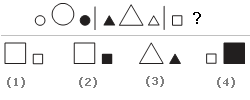In each segment, the shapes are the same, but the one in the middle is larger than the two on either side. Also, one of the figures is shaded and that this shading alternates between 1st right and then left. Thus in the 3rd segment, there will be two small squares with a big square between them and the right small square will be blackened. Thus, the correct option is (2).

Question: Look carefully at the sequence of symbols to find the missing pattern.

Solution: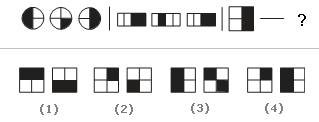In each of the segments, the figures alternate between one-half and one-fourth shaded on the opposite sides. Thus, to complete the last segment, there should be one square that is one-fourth shaded and another that is half-shaded on the left side. Thus, the correct option is (4).

Tip #3: Eliminate the incorrect choices

Question: Look carefully at the sequence of symbols to find the missing pattern.

Solution: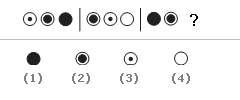Since no 2 figures in the same segment are the same, Options (1) and (2) are eliminated. Now, the shading inside the circles gradually increases or decreases. Thus, the correct option is (3).

Question: Look at the following series and fill in the blank. JAK, KBL, LCM, MDN, _____

A. OEP    B. NEO    C. MEN    D.PFQ

Solution:

The first letter of each term follows the series J, K, L, M and N. This eliminates choices A, C, and D. Thus, the correct answer is B.

Question: Look carefully at the sequence of symbols to find the missing pattern.

Solution: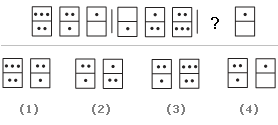In all these segments, the count of dots in the bottom box of the previous set equals the count of dots in the top box of the current set. This eliminates options (2), (3) and (4). Thus, the correct option is (1).